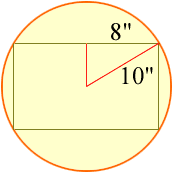Quandaries and Queries Name: Arthur Who is asking: Student Level: Secondary Question: How do I go about solving the following problem: What is the width of the largest rectangle with a length of 16 inches you can cut from a circular piece of cardboard having a 10 inch radius? Hi Arthur, Draw a diagram with the largest possible rectangle on the disk of cardboard.Draw a line from the center of the circle to the center of one of the sides of the rectangle, and another line from center of the circle to a vertex of the rectangle to form a triangle as in the diagram above. The triangle has a right angle, one side is of length 8" and the hypotenuse is of length 10". What is the length of the other side? Penny Go to Math Central